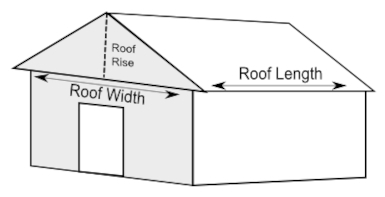# Roofing Project Calculations: Essential Formulas

Roofing projects are crucial aspects of construction, requiring accurate calculations to ensure structural integrity, material estimation, and budgeting. Whether you're a seasoned contractor or a DIY enthusiast, understanding the calculations involved in roofing projects is essential.

In this article, we will delve into the fundamental construction calculations required for successful roofing projects. We'll cover key concepts such as roof area determination, pitch calculations, material estimation, and load-bearing capacity assessments.## Roof Area Calculation

The first step in any roofing project is to accurately calculate the roof area. This calculation forms the foundation for determining material quantities and costs. The formula to calculate the roof area depends on the shape of the roof:

• Flat or Low-Slope Roof: Roof area = Length x Width
• Gable Roof: Roof area = (0.5 x Length) x Width
• Hip Roof: Roof area = 1.15 x (0.5 x A x B + 0.5 x B x C)

Where A, B, and C are the lengths of the three sides of the hip roof. Ensure all measurements are in the same unit, typically feet or meters.

For example, consider a gable roof with a length of 40 feet and a width of 30 feet:

```            Roof area = (0.5 x 40) x 30
= 600 square feet
```

## Pitch Calculation

The roof pitch indicates the steepness of the roof and is crucial for choosing appropriate roofing materials and designing proper drainage systems. It's usually represented as a ratio of vertical rise to horizontal run.

The pitch can be calculated using the formula:

```            Pitch = Rise / Run
```

Where the rise is the vertical measurement from the top of the wall plate to the highest point of the roof, and the run is the horizontal distance from the wall plate to the roof ridge. Pitch is often expressed as a ratio like 4:12 or as a percentage by multiplying the ratio by 100.

For instance, if the rise is 8 feet and the run is 24 feet, the pitch can be calculated as:

```            Pitch = 8 / 24
= 1/3
≈ 33.33%
```

## Material Estimation

Accurate material estimation ensures that you have the right amount of roofing materials, preventing delays and cost overruns. The quantity of materials needed depends on the roof area, pitch, and the type of roofing material being used.

The basic formula for material estimation is:

```            Material Quantity = Roof Area / Coverage Area per Unit
```

Where the coverage area per unit is the area covered by a single unit of the roofing material, taking into account overlaps and wastage.

For example, if you're using asphalt shingles that cover 100 square feet per bundle, and your roof area is 1200 square feet:

```            Material Quantity = 1200 / 100
= 12 bundles
```

Before beginning a roofing project, it's crucial to assess the load-bearing capacity of the roof structure. This ensures that the roof can support the weight of the roofing materials, snow, and other potential loads. The load-bearing capacity can be calculated using the formula:

```        Load Capacity = Dead Load + Live Load
```

Where the dead load includes the weight of the roof itself, and the live load includes temporary loads like snow accumulation or maintenance personnel.

For example, if the dead load is 5 psf (pounds per square foot) and the live load is 20 psf, the load-bearing capacity would be:

```        Load Capacity = 5 + 20
= 25 psf
```

## Truss Calculation for Pitched Roofs

Pitched roofs often require truss systems to support the roof's weight and maintain its structural integrity. Calculating the truss dimensions is essential for proper installation. The span, rise, and run of the truss can be calculated using trigonometric formulas:

```        Span = 2 * (Rise / tan(θ))
Run = 2 * (Rise / sin(θ))
```

Where θ is the angle of the roof pitch.

For instance, if the roof pitch is 30 degrees and the rise is 8 feet:

```        Span = 2 * (8 / tan(30°))
≈ 27.71 feet
Run = 2 * (8 / sin(30°))
≈ 32 feet
```

## Calculating Roof Slope Factor

The roof slope factor is used to convert the horizontal area of the roof to the actual surface area. It accounts for the increased surface area due to the slope of the roof. The formula to calculate the slope factor is:

```        Slope Factor = √(1 + Rise^2 / Run^2)
```

Using the previous example with a rise of 8 feet and a run of 24 feet:

```        Slope Factor = √(1 + 8^2 / 24^2)
≈ 1.333
```

## Conclusion

Accurate calculations are the backbone of successful roofing projects. From determining roof area and pitch to estimating material quantities and load-bearing capacity, these calculations guide construction professionals and enthusiasts alike in making informed decisions. Whether you're repairing a small section or installing a new roof, mastering these construction calculations is essential for a job well done.

Remember, while these formulas provide a solid foundation, consulting with structural engineers and roofing experts is advisable for complex projects to ensure safety and compliance with local building codes.